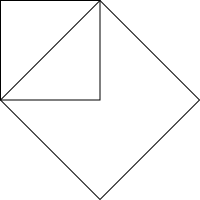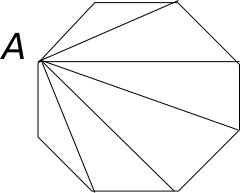#Solutions for Practice Test 3, The Official SAT Study Guide, Section 5

Math Lair Home > Test Preparation > Solutions for Practice Test 3, The Official SAT Study Guide, Section 5
SAT Practice Test Solutions:
2014–15 SAT Practice Test
2013–14 SAT Practice Test
The Official SAT Study Guide, second edition
• Practice Test 1: Sections 3, 7, 8.
• Practice Test 2: Sections 2, 5, 8.
• Practice Test 3: Sections 2, 5, 8.
• Practice Test 4: Sections 3, 6, 9.
• Practice Test 5: Sections 2, 4, 8.
• Practice Test 6: Sections 2, 4, 8.
• Practice Test 7: Sections 3, 7, 9.
• Practice Test 8: Sections 3, 7, 9.
• Practice Test 9: Sections 2, 5, 8.
• Practice Test 10: Sections 2, 5, 8.
SAT Math Tips

Here are solutions for section 5 of the third practice test in The Official SAT Study Guide, second edition, found on pages 525–530. The following solutions illustrate faster, less formal methods that may work better than formal methods on a fast-paced test such as the SAT. To learn more about these methods, see my e-book Succeeding in SAT Mathor the SAT math tips page.

1. If Dave owns 44 comic books, then Fred and Norman own a total of 84 comic books. So, the average number of comic books owned by the two is 84 ÷ 2 = 42. Select (A) 42.
• Estimate the answer: The x-coordinate of the answer must be zero. P appears to be as far away from the origin as (6, 0) is, so the y-coordinate is about 6. So, P is something like (0, 6).
• Look at the answer choices: The only answer choice anywhere near our estimate is (B) (0, 6). Select that answer.
• Try a special case: Say that two copies were made (n = 2). The cost would be \$1.00 + 2(\$0.10) = \$1.20.
• Look at the answer choices: See which choice evaluates to \$1.20 when n = 2:
• (A) evaluates to 0.9(2) = \$1.80
• (B) evaluates to 1.1(2) = \$2.20
• (C) evaluates to 1 + 10(2) = \$21.
• (D) evaluates to 1 + 0.1(2) = \$1.20.
• (E) evaluates to 1 + 0.1 + 2 = \$3.10.
The only answer that matches is (D) 1.00 + 0.10n. Select that answer.
• Read the question and understand what it is asking: A letter pair with two as has a value of 2. A letter pair with one a has a value of 1. In other words, the value of the letter pair is just the number of as in it.
• So, the answer is equal to the number of as. Counting them, there are 7. Select (B) 7.
2. Solution 1:
• Estimate the answer: If the diagonal of the square is 4, the side length of the square must be less, say around 3. So, the area of the square would be somewhere around 9.
• Look at the answer choices: (C), (D), and (E) are definitely too large. (A) 8 is close to our estimate, but you may want to ensure that the answer is not (B).
• First, find the side length of the square. The square is made up of two 45°-45°-90° right triangles. From the Reference Information, the other two sides of the triangle must be 4⁄√2.
• The area of the square is (4⁄√2)² = 16⁄2 = 8. Select (A) 8.
Solution 2:
•Draw a diagram: On the given diagram, draw a square with AC being one of the sides, as shown on the right.
• Since the side length of the square just drawn is 4, its area is equal to 16. Now, the area of the square is equal to four of the small triangles, so the triangle has area 4. Square ABCD is made up of two triangles, so its area is 2 × 4 = 8. Select (A) 8.
3. Solution 1: If something is directly proportional to 1⁄x², it is inversely proportional to x². Since x is inversely proportional to y, x² is inversely proportional to y². Select (E) y².

Solution 2:
• Try a special case: Pick a few values for x and y such that x is inversely proportional to y. You might come up with a table such as the following:
xy
121
62
43
34
• Add a column to your table for 1⁄x²:
xy1⁄x²
1211⁄144
621⁄36
431⁄16
341⁄9

When x goes from 12 to 6, 1⁄x² increases by a factor of 4. When x goes from 12 to 6, y goes from 1 to 2, which is an increase by a factor of 2. y² would increase by a factor of 4. You can try a few more numbers and verify that the correct answer is (E) y².

• Draw a diagram: Draw an eight-sided polygon, label one of the vertices A, and draw all possible diagonals:• Count the number of triangles in the diagram: There are six triangles. Select (C) Six.
• Expand the left hand side of the equation:
x² − (8 + k)x + 8k = x² −5kx + m
• Now, if the equation is true for all values of x, then the coefficient of x must be the same on both sides of the equation, as must the constant term. Looking at the coefficient of x:
−(8 + k) = −5k
8 = 4k
k = 2
• To find the value of m, set the constant terms equal to one another, and set k = 2:
8k = m
8(2) = m
m = 16
Select (B) 16.
4. This is a fairly simple proportion question. If the bird flew 62 miles in 4 hours, it flew 62 × ¾ = 46.5 miles in 3 hours. Enter 46.5.
5. Draw a diagram: Draw a diagram similar to the following:Now, PQ, PR, PS, and PT are all radii of the circle. Since the radius of the circle is 1, the sum of these four lengths is 4.
6. Solution 1: If a and b are positive integers, so is ab. All positive integers are divisible by 1, so regardless of what the value of ab is, a possible value for a is 1. Enter 1.

Solution 2: 10,000 = 104, so ab = 4. By trial and error, find values for a and b. For example, a = 2, b = 2. Enter 2.
7. When x = 5 and y = −1:
2(5) − 3(−1) = c
c = 13
Enter 13.
• Draw a diagram: The graph is given, but draw the line x = y through the graph, representing numbers where the number of males and females are equal.
• To find the value where the absolute value of the difference between the number of males and females is greatest, find the point that is the furthest away from the point you drew. This point is 1992.
8. Convert the sentence into an equation:
SentenceEquation
five5
times·
a numberx
is the same as=
the numberx
five5
This results in:
5x = x + 5
4x = 5
x = 5⁄4
Enter 5/4.
• Draw a diagram: The diagram is drawn, but label it according to the question. Since OB bisects ∠AOD, and ∠ AOB = x°, then ∠BOD = x°. Since OD bisects ∠AOF and ∠AOD = x° + x° = 2x°, then ∠DOF = 2x°.
• To find what we're looking for, look at ∠BOE as a sum and/or difference of angles that we know:
BOE = ∠BOD + ∠DOF − ∠EOF
= x° + 2x° −y°
= 40° + 2(40)° − 30°
= 90°
Enter 90.
9. Since there are 1 1s, 2 2s, 3 3s, and so on, there will be 1 + 2 + 3 + 4 + 5 + 6 + 7 + 8 + 9 + 10 + 11 terms in the sequence before a 12 appears. Adding these terms up (you may be able to find a shortcut way of doing so) results in 66. There is a little trick here, though. The 12 appears after these 66 terms, so it is the 67th term. Enter 67.
10. The pattern in the paper repeats every 4 inches. Every 4 inches, the length of the notched edge increases by 3 + 1 + 1 = 5 inches. So, after 80 inches, the length will be (80 ÷ 4) × 5 = 100.
• If the square has an area of 64, the length of its side is 8. Looking at the graph, on the coordinate plane, its y-coordinates range from 0 to 8, and its x-coordinates range from −4 to 4.
• So, the coordinate of R is (4, 8).
• (4, 8) is a point on the parabola, so substitute x = 4, y = 8 into y = ax²
8 = a(4)² 8⁄16 = a a = ½
Enter 1/2.